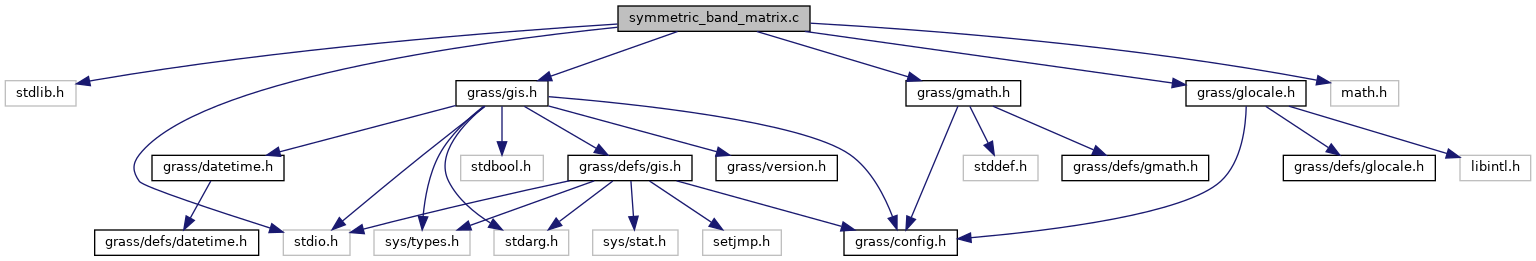GRASS GIS 7 Programmer's Manual  7.9.dev(2021)-e5379bbd7
symmetric_band_matrix.c File Reference
`#include <stdlib.h>`
`#include <stdio.h>`
`#include <math.h>`
`#include <grass/gis.h>`
`#include <grass/gmath.h>`
`#include <grass/glocale.h>`
Include dependency graph for symmetric_band_matrix.c:Go to the source code of this file.

## Functions

double ** G_math_matrix_to_sband_matrix (double **A, int rows, int bandwidth)
Convert a symmetrix matrix into a symmetric band matrix. More...

double ** G_math_sband_matrix_to_matrix (double **A, int rows, int bandwidth)
Convert a symmetric band matrix into a symmetric matrix. More...

void G_math_Ax_sband (double **A, double *x, double *y, int rows, int bandwidth)
Compute the matrix - vector product of symmetric band matrix A and vector x. More...

## ◆ G_math_Ax_sband()

 void G_math_Ax_sband ( double ** A, double * x, double * y, int rows, int bandwidth )

Compute the matrix - vector product of symmetric band matrix A and vector x.

This function is multi-threaded with OpenMP and can be called within a parallel OpenMP region.

y = A * x

Parameters
 A (double **) x (double) *) y (double * ) rows (int) bandwidth (int)
Returns
(void)

Definition at line 123 of file symmetric_band_matrix.c.

## ◆ G_math_matrix_to_sband_matrix()

 double** G_math_matrix_to_sband_matrix ( double ** A, int rows, int bandwidth )

Convert a symmetrix matrix into a symmetric band matrix.

```Symmetric matrix with bandwidth of 3

5 2 1 0
2 5 2 1
1 2 5 2
0 1 2 5

will be converted into the symmetric band matrix

5 2 1
5 2 1
5 2 0
5 0 0```
Parameters
 A (double**) the symmetric matrix rows (int) bandwidth (int)
Returns
B (double**) new created symmetric band matrix

Definition at line 34 of file symmetric_band_matrix.c.

## ◆ G_math_sband_matrix_to_matrix()

 double** G_math_sband_matrix_to_matrix ( double ** A, int rows, int bandwidth )

Convert a symmetric band matrix into a symmetric matrix.

```Such a symmetric band matrix with banwidth 3

5 2 1
5 2 1
5 2 0
5 0 0

Will be converted into this symmetric matrix

5 2 1 0
2 5 2 1
1 2 5 2
0 1 2 5```
Parameters
 A (double**) the symmetric band matrix rows (int) bandwidth (int)
Returns
B (double**) new created symmetric matrix

Definition at line 80 of file symmetric_band_matrix.c.